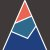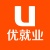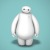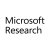This paper proposes a cascading failure mitigation strategy based on Reinforcement Learning (RL) method. Firstly, the principles of RL are introduced. Then, the Multi-Stage Cascading Failure (MSCF) problem is presented and its challenges are investigated. The problem is then tackled by the RL based on DC-OPF (Optimal Power Flow). Designs of the key elements of the RL framework (rewards, states, etc.) are also discussed in detail. Experiments on the IEEE 118-bus system by both shallow and deep neural networks demonstrate promising results in terms of reduced system collapse rates.The approximate degree of a Boolean function f is the least degree of a real polynomial that approximates f pointwise to error at most 1/3. Approximate degree is known to be a lower bound on quantum query complexity. We resolve or nearly resolve the approximate degree and quantum query complexities of the following basic functions: $\bullet$ $k$-distinctness: For any constant $k$, the approximate degree and quantum query complexity of $k$-distinctness is $\Omega(n^{3/4-1/(2k)})$. This is nearly tight for large $k$ (Belovs, FOCS 2012). $\bullet$ Image size testing: The approximate degree and quantum query complexity of testing the size of the image of a function $[n] \to [n]$ is $\tilde{\Omega}(n^{1/2})$. This proves a conjecture of Ambainis et al. (SODA 2016), and it implies the following lower bounds: $-$ $k$-junta testing: A tight $\tilde{\Omega}(k^{1/2})$ lower bound, answering the main open question of Ambainis et al. (SODA 2016). $-$ Statistical Distance from Uniform: A tight $\tilde{\Omega}(n^{1/2})$ lower bound, answering the main question left open by Bravyi et al. (STACS 2010 and IEEE Trans. Inf. Theory 2011). $-$ Shannon entropy: A tight $\tilde{\Omega}(n^{1/2})$ lower bound, answering a question of Li and Wu (2017). $\bullet$ Surjectivity: The approximate degree of the Surjectivity function is $\tilde{\Omega}(n^{3/4})$. The best prior lower bound was $\Omega(n^{2/3})$. Our result matches an upper bound of $\tilde{O}(n^{3/4})$ due to Sherstov, which we reprove using different techniques. The quantum query complexity of this function is known to be $\Theta(n)$ (Beame and Machmouchi, QIC 2012 and Sherstov, FOCS 2015). Our upper bound for Surjectivity introduces new techniques for approximating Boolean functions by low-degree polynomials. Our lower bounds are proved by significantly refining techniques recently introduced by Bun and Thaler (FOCS 2017).In caching system, we prefer to design a coded caching scheme with the rate $R$ and the packet number $F$ as small as possible since the efficiency of transmission in the peak traffic times increases with the decreasing of $R$ and the realizing complexity increases with the increasing of $F$. In this paper, we first introduce a framework for constructing coded caching schemes, which can represent almost all of the previously known schemes. Based on this framework, we obtain a new scheme, which generalizes the schemes constructed by Shangguan et al. (IEEE Trans. Inf. Theory, 64, 5755-5766, 2018) and Yan et al. ( IEEE Commun. Lett., 22, 236-239, 2018) and has better performance compared with these two schemes since it has advantages on the user number, the coded gains and the flexible memory size. Then the relationships between a coded caching scheme and orthogonal array, covering array are derived respectively. Consequently a tight lower bound on the packet number $F$ is derived since the packet number of the schemes constructed by Yan et al. (IEEE Trans. Inf. Theory 63, 5821-5833, 2017) gets this lower bound. Finally based on orthogonal array, we construct a new scheme which has the same user number, memory size and transmission rate as the scheme constructed by Shangguan et al. (IEEE Trans. Inf. Theory, 64, 5755-5766, 2018) but has smaller packet number. Based on MDS codes, more classes of schemes are obtained using our framework, in which the distribution of the coded gain is equivalent to solving a linear system of equations.The peak-to-average-power ratio (PAPR) of the frequency domain multiplexed wake-up signals (WUSs) specified in IEEE P802.11ba can be very large and difficult to manage since it depends on the number and allocation of the active channels, and the data rate on each channel. To address this issue, we propose a transmission scheme based on complementary sequences (CSs) for multiple WUSs multiplexed in the frequency domain. We discuss how to construct CSs compatible with the framework of IEEE P802.11ba by exploiting a recursive Golay complementary pair (GCP) construction to reduce the instantaneous power fluctuations in time. We compare the proposed scheme with the other options under a non-linear power amplifier (PA) distortion. Numerical results show that the proposed scheme can lower the PAPR of the transmitted signal in frequency division multiple access (FDMA) scenarios more than 3 dB and yields a superior error rate performance under severe PA distortion.Wi-Fi was originally designed to provide broadband wireless Internet access for devices which generate rather heavy streams. And Wi-Fi succeeded. The coming revolution of the Internet of Things with myriads of autonomous devices and machine type communications (MTC) traffic raises a question: can the Wi-Fi success story be repeated in the area of MTC? Started in 2010, IEEE 802.11 Task Group ah (TGah) has developed a draft amendment to the IEEE 802.11 standard, adapting Wi-Fi for MTC requirements. The performance of novel channel access enhancements in MTC scenarios can hardly be studied with models from Bianchi's clan, which typically assume that traffic load does not change with time. This paper contributes with a pioneer analytical approach to study Wi-Fi-based MTC, which can be used to investigate and customize many mechanisms developed by TGah.During the last decade, the number of devices connected to the Internet by Wi-Fi has grown significantly. A high density of both the client devices and the hot spots posed new challenges related to providing the desired quality of service in the current and emerging scenarios. To cope with the negative effects caused by network densification, modern Wi-Fi is becoming more and more centralized. To improve network efficiency, today many new Wi-Fi deployments are under control of management systems that optimize network parameters in a centralized manner. In the paper, for such a cloud management system, we develop an algorithm which aims at maximizing energy efficiency and also keeps fairness among clients. For that, we design an objective function and solve an optimization problem using the branch and bound approach. To evaluate the efficiency of the developed solution, we implement it in the NS-3 simulator and compare with existing solutions and legacy behavior.Recently, a medical privacy protection scheme (MPPS) based on DNA encoding and chaos was proposed in [IEEE Trans. Nanobioscience, vol. 16, pp. 850--858, 2017]. This paper reports some properties of MPPS and proposes a chosen-plaintext attack on it. In addition, the other claimed superiorities are questioned from the viewpoint of modern cryptography. Both theoretical analysis and experimental results are provided to support the feasibility of the attack and other security defects. The proposed cryptanalysis will promote proper application of DND encoding in protecting multimedia data including DICOM image.This work proposes a moving target defense (MTD) strategy to detect coordinated cyber-physical attacks (CCPAs) against power grids. A CCPA consists of a physical attack, such as disconnecting a transmission line, followed by a coordinated cyber attack that injects false data into the sensor measurements to mask the effects of the physical attack. Such attacks can lead to undetectable line outages and cause significant damage to the grid. The main idea of the proposed approach is to invalidate the knowledge that the attackers use to mask the effects of the physical attack by actively perturbing the grid's transmission line reactances using distributed flexible AC transmission system (D-FACTS) devices. We identify the MTD design criteria in this context to thwart CCPAs. The proposed MTD design consists of two parts. First, we identify the subset of links for D-FACTS device deployment that enables the defender to detect CCPAs against any link in the system. Then, in order to minimize the defense cost during the system's operational time, we use a game-theoretic approach to identify the best subset of links (within the D-FACTS deployment set) to perturb which will provide adequate protection. Extensive simulations performed using the MATPOWER simulator on IEEE bus systems verify the effectiveness of our approach in detecting CCPAs and reducing the operator's defense cost.Performance improvement has been among the foci of all previous amendments of IEEE 802.11 protocol. In addition, the draft high efficiency (HE) amendment IEEE 802.11ax, proposed by TGax, aims at increasing network performance. One of the main obstacles to improving spectral and power efficiency is the presence of hidden terminals which degrade throughput, in particular in uplink transmission. IEEE 802.11ax does provide mechanisms such as trigger based uplink transmission that mitigate this degradation to some extent, but are incapable of eliminating it, esp. at high arrival rates. To combat the hidden terminal problem, we propose to increase the carrier sensing threshold (CSTH) of STAs during association with an HE access point. Our results confirm that the proposed mechanism can lead to significant reduction of collision probability in uplink transmission.Minimal linear codes have interesting applications in secret sharing schemes and secure two-party computation. This paper uses characteristic functions of some subsets of $\mathbb{F}_q$ to construct minimal linear codes. By properties of characteristic functions, we can obtain more minimal binary linear codes from known minimal binary linear codes, which generalizes results of Ding et al. [IEEE Trans. Inf. Theory, vol. 64, no. 10, pp. 6536-6545, 2018]. By characteristic functions corresponding to some subspaces of $\mathbb{F}_q$, we obtain many minimal linear codes, which generalizes results of [IEEE Trans. Inf. Theory, vol. 64, no. 10, pp. 6536-6545, 2018] and [IEEE Trans. Inf. Theory, DOI: 10.1109/TIT.2019.2918537]. Finally, we use characteristic functions to present a characterization of minimal linear codes from the defining set method.

Top